# 25+ Depreciation Schedule Template Excel Free to Use

Depreciation Schedule Template: Over time, an asset wouldn’t have the same value anymore like when it was new. Thus, in the business, this impairment should get counted and calculate over the asset. To do that, you don’t need to wait until for the asset’ value to decrease on its own because you can count it by using an accounting tool. It called depreciation, which is a tool for spreading valuable assets’ cost over a period of time in accounting. That’s why you need for depreciation schedule template excel.

Contents

## Methods on How to Make Depreciation Schedule

It would be easier to calculate depreciation schedule template excel since spreadsheet would help you in making the calculation. Not to mention, it has a wide range of rows and columns. That way, you can put a long list of your business assets over a period of times. While making for the depreciation schedule, there are three methods in that you can use, they are:

### Straight Line Method

The first method that most people often use in making depreciation schedule sample is a straight line. By using this method, you count the value of the assets in a straight line from its best condition to its end time. Usually, you use this method for degradation asset such as a production machine. To do this method, here are what you have to do:

• First, you have to determinate the asset’s salvage value (how much you could get from it if you sell it). In this step, you have to determinate on the price when you purchase this and how long it would last in your possession (in years).
• The second step, you have to subtract the nominal on the purchase value over its salvage value.
• For the last step, you have to divide the difference of the salvage value from the purchase value by its expecting life span. That way, you would get the annual depreciation and put them in the span of years in a straight line.

### Production Units Method

The second method is by counting on the production units you expect to produce. Thus, the method accentuated on the number of the production process you could get. This method is better for an extraordinary asset that only used for a special occasion like for special production. Here below is how you do this method:

• You have to determinate on the purchase value and salvage value, then estimate the exact number of production you expect over the years (annually).
• Make the subtraction from the salvage value over the purchase value.
• Lastly, divide the total of the year production number over the total amount of the production through the years of the assets life span. Then you have to multiply the result with the second step result.

### Declining Balance Method

The last method is by counting on the decline of the production balance, not only over the asset but also for the market value. Usually, this used for a fast-paced asset like computer and gadget. Here are the steps on how to do this method:

• Count on the purchase price, salvage value, and the expected life (in years). Later you should also count on the market value for the asset through the years. Then use the market value to subtract the value from the previous year to get the depreciation value.
• Divide 1.5 by the life span you expect from the asset, and then multiply the result by the market value of the year.
• For the previous step, you can divide 2 (not 1.5) by the expected year for the double declining balance method.

## Depreciation Schedule Template Excel Free to Use

Making the depreciation schedule template is not an easy thing to do for most people. The reason is that not everyone familiar with the accounting method. But this step is important to in calculating the asset value over time. Thus, for a shortcut, you can use these examples of depreciation template schedule excel attached here for free.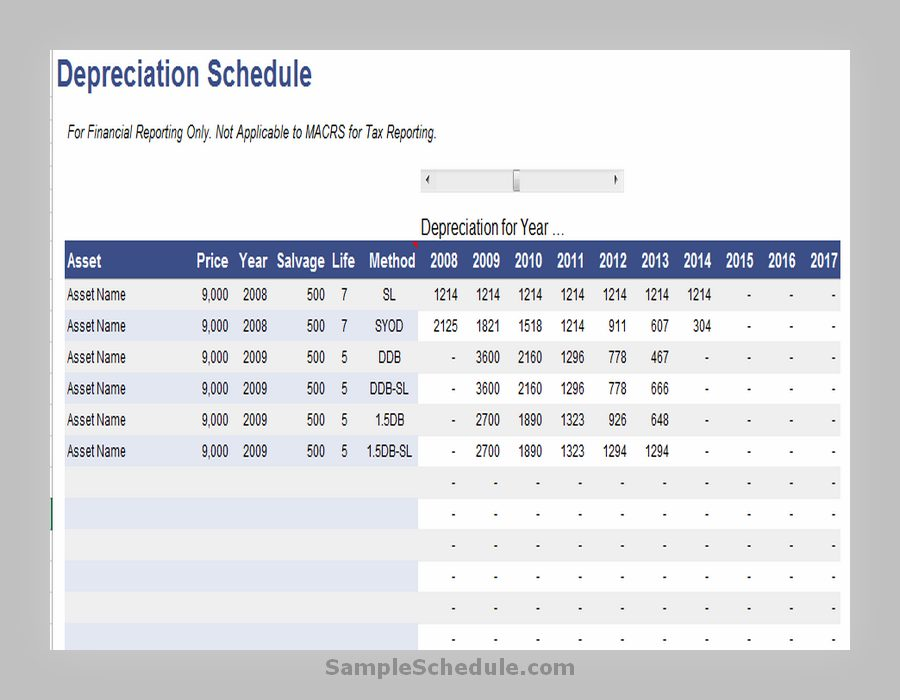Depreciation Schedule Template 01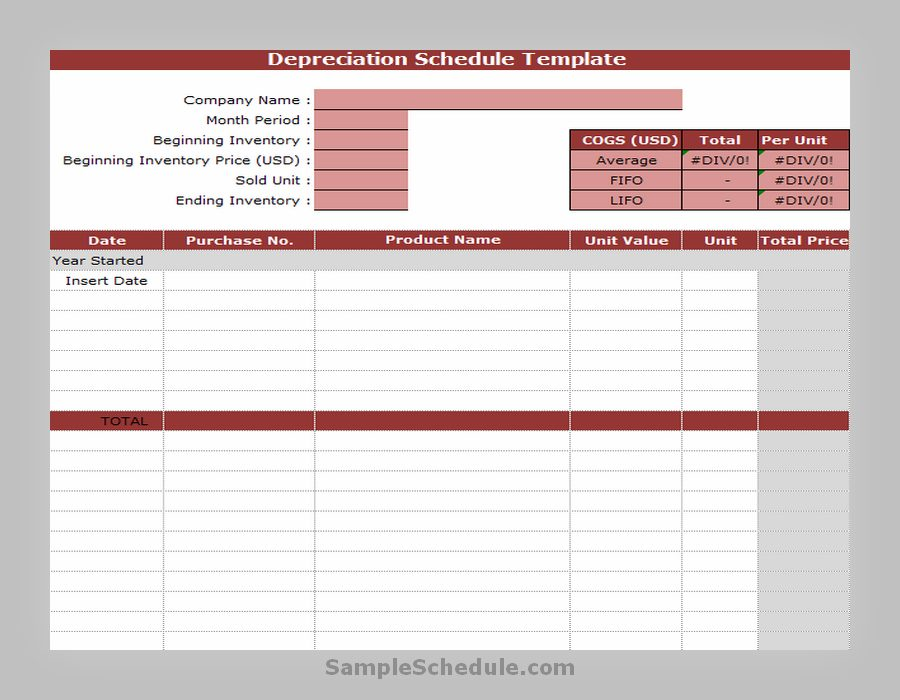Depreciation Schedule Template 20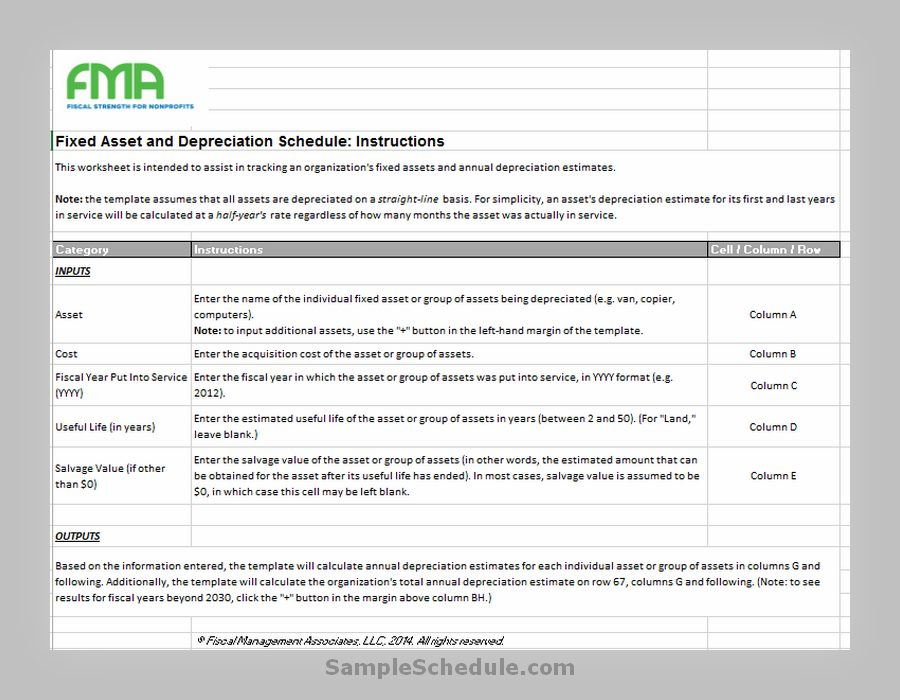Depreciation Schedule Template 02Depreciation Schedule Template 03Depreciation Schedule Template 04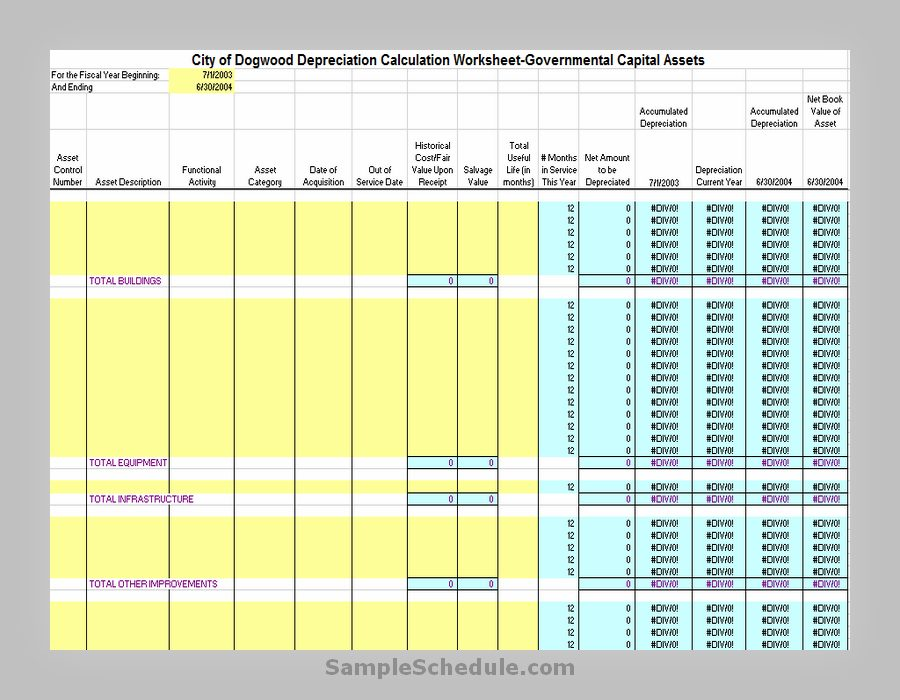Depreciation Schedule Template 05Depreciation Schedule Template 06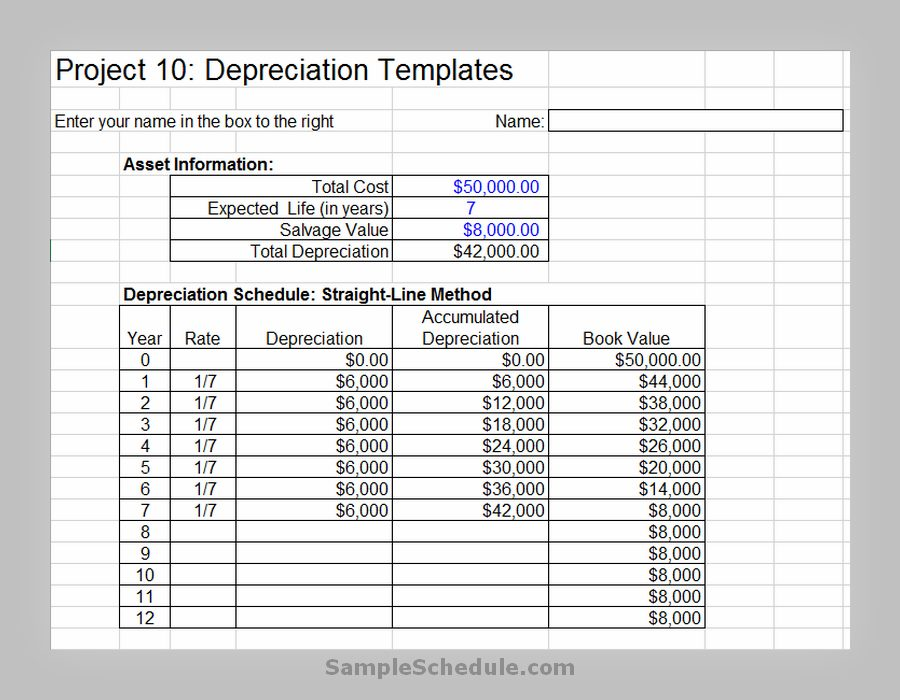Depreciation Schedule Template 07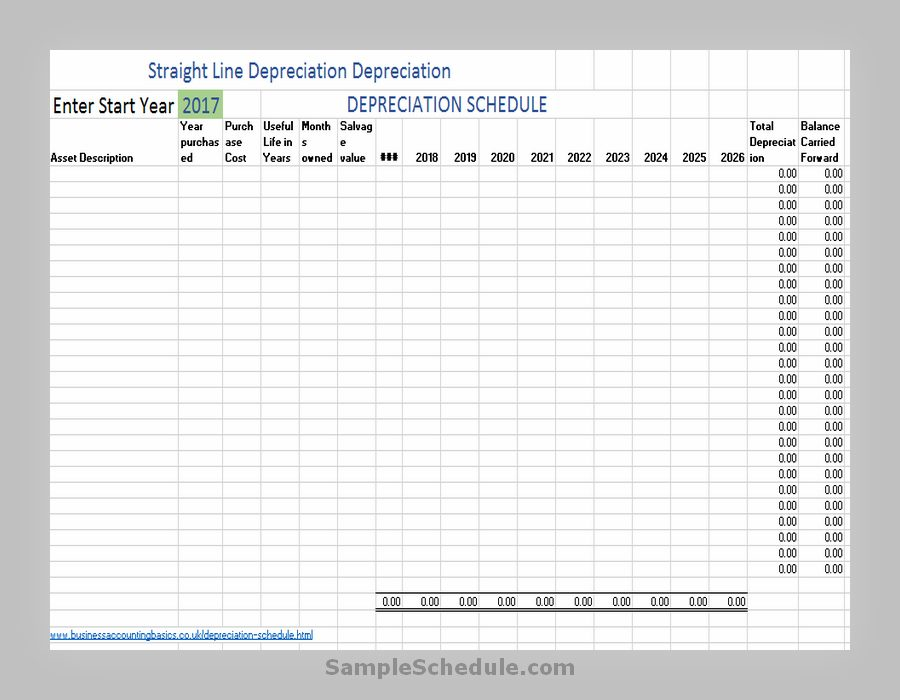Depreciation Schedule Template 08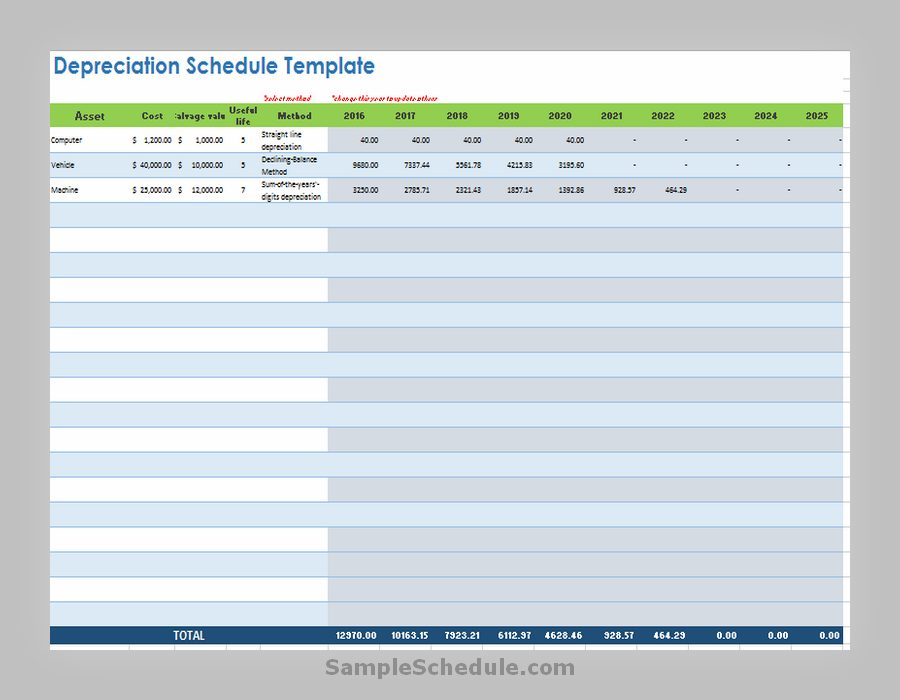Depreciation Schedule Template 09Depreciation Schedule Excel Template 10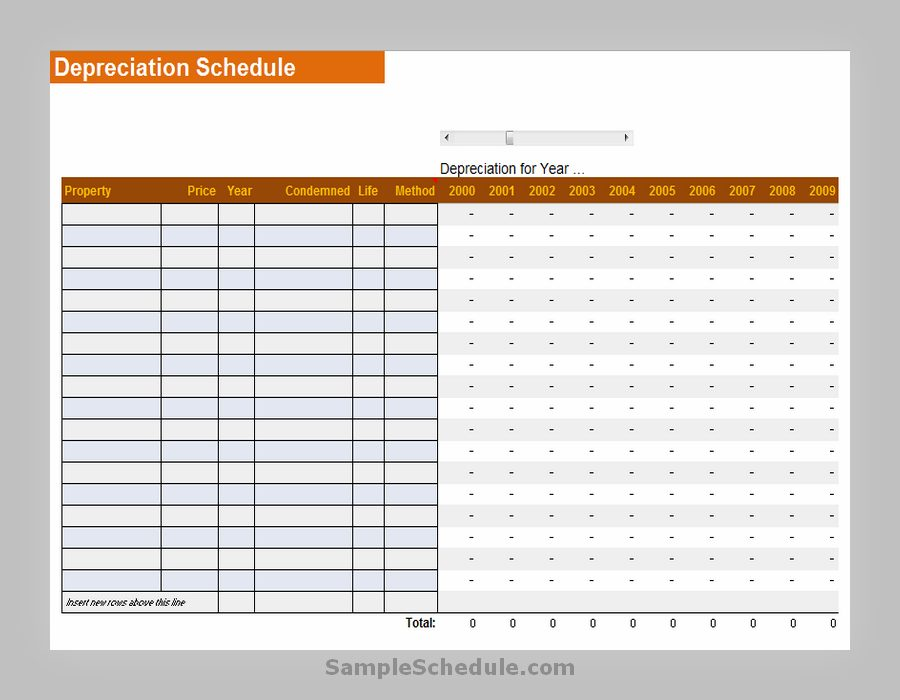Depreciation Schedule Excel Template 11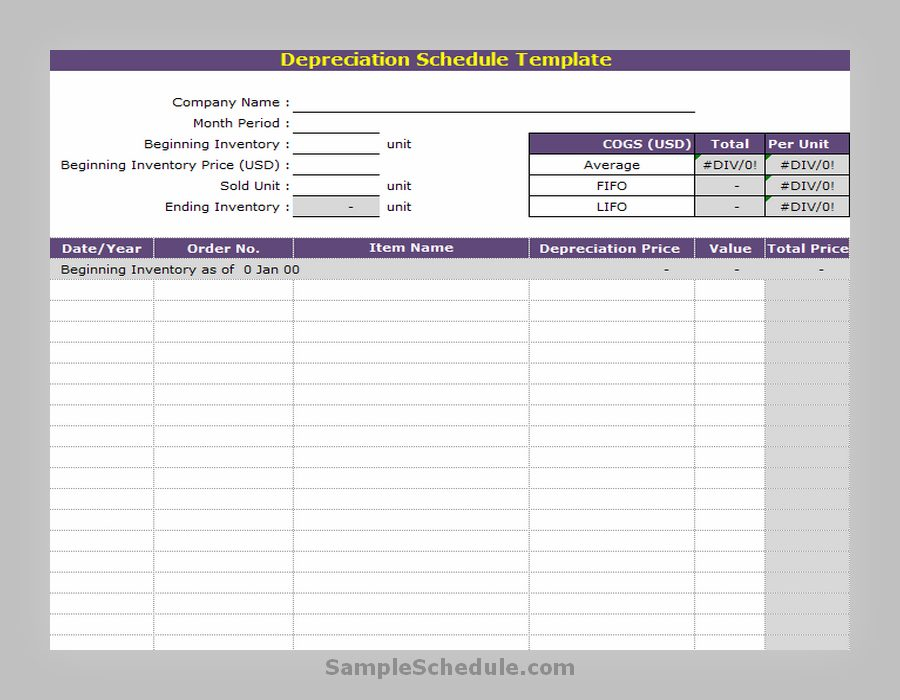Depreciation Schedule Excel Template 12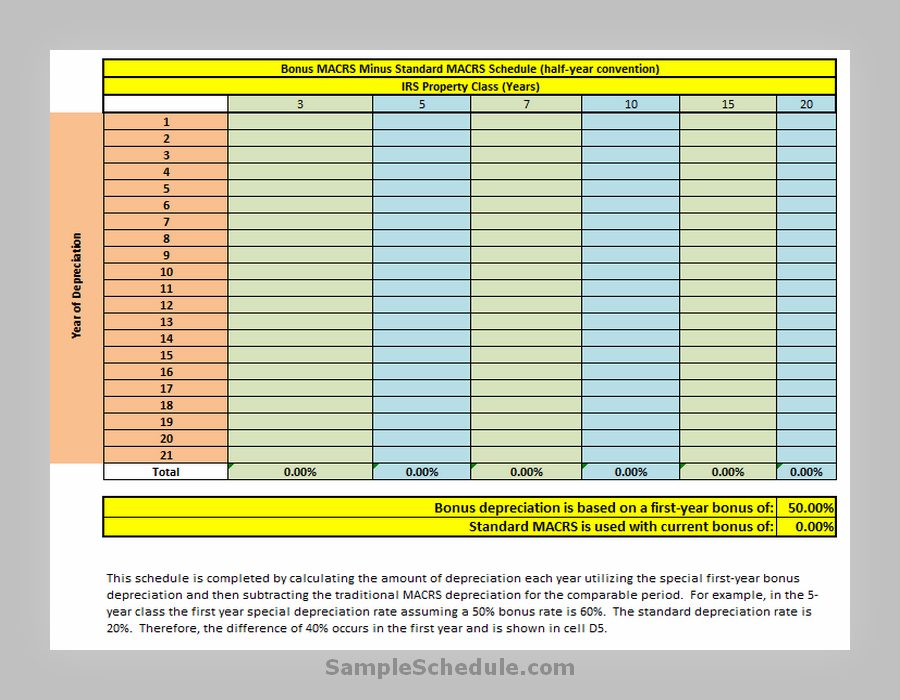Depreciation Schedule Excel Template 13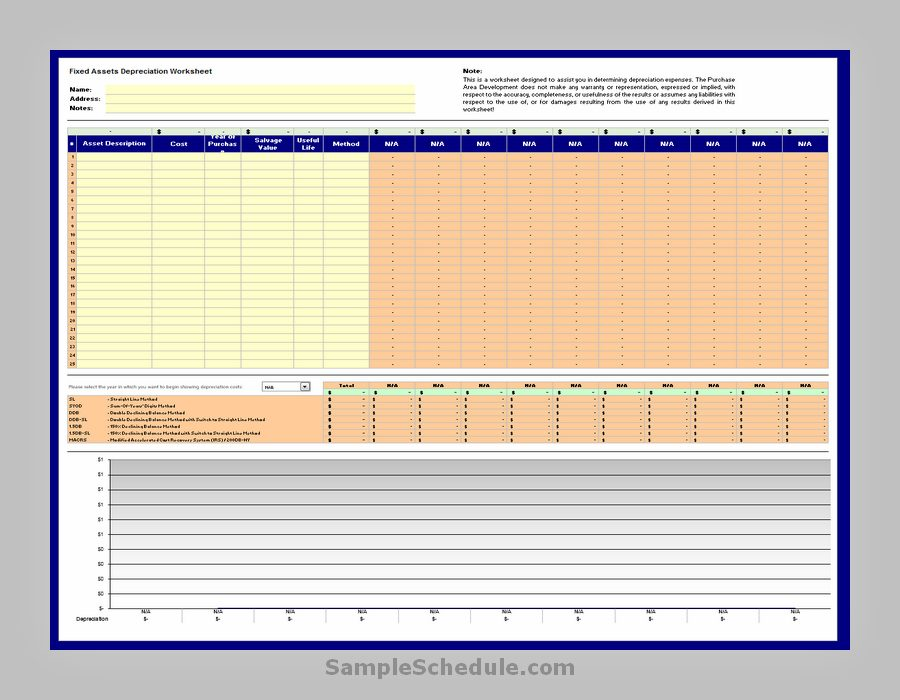Depreciation Schedule Excel Template 14Depreciation Schedule Excel Template 15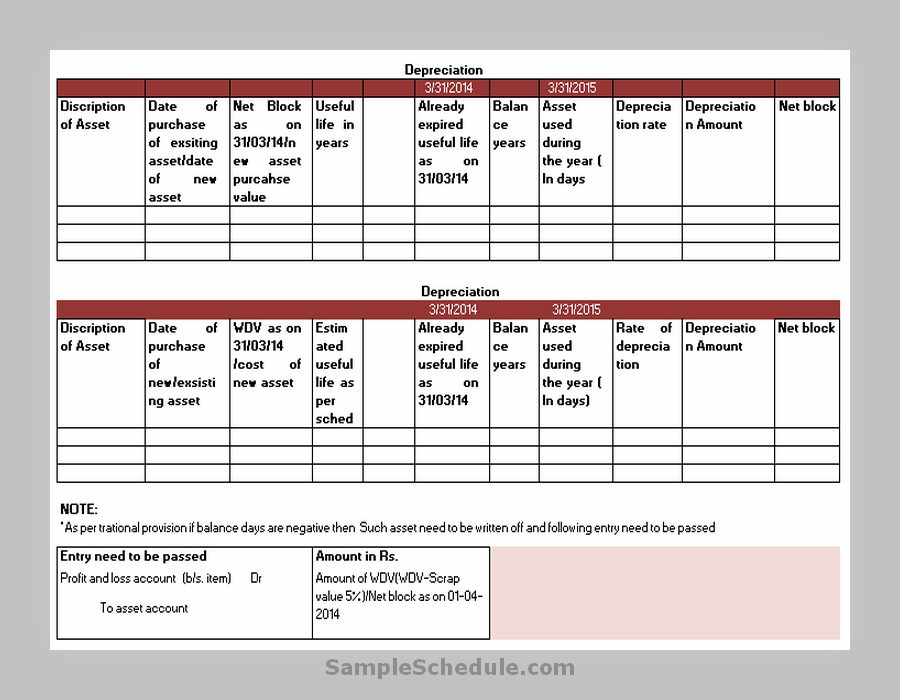Depreciation Schedule Excel Template 16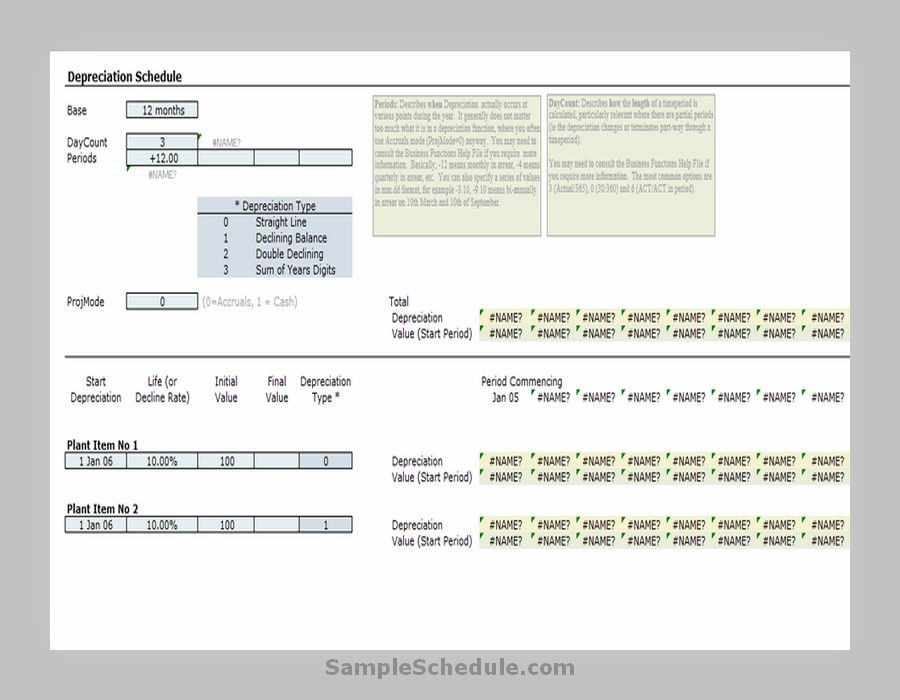Depreciation Schedule Excel Template 17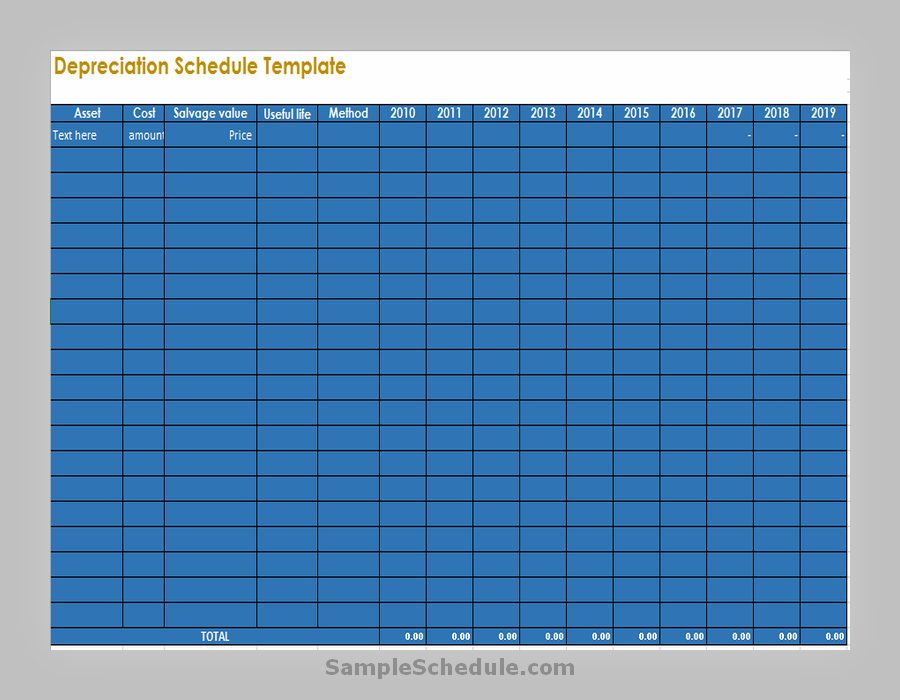Depreciation Schedule Excel Template 18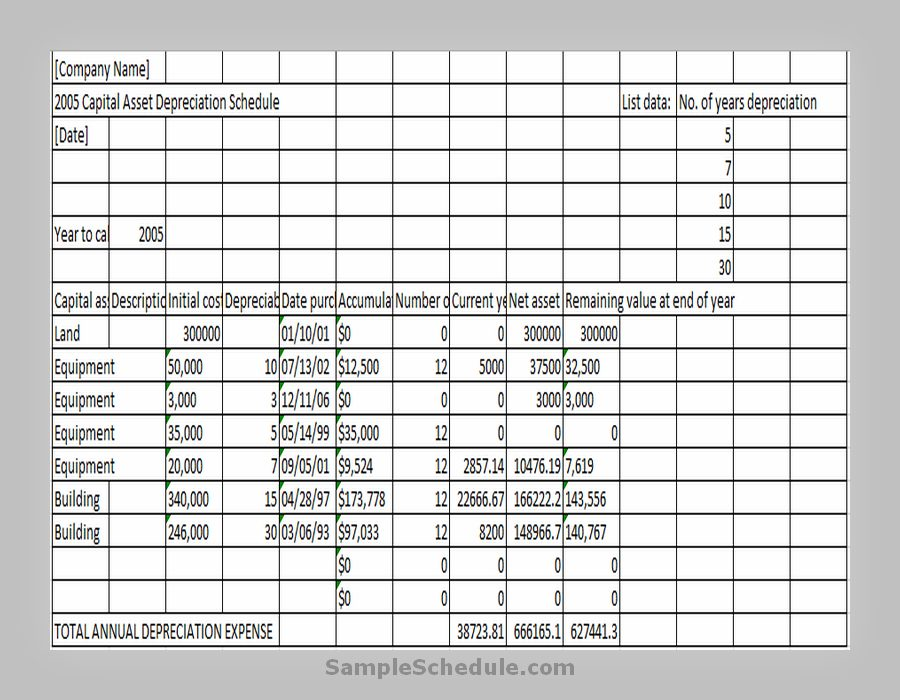Depreciation Schedule 19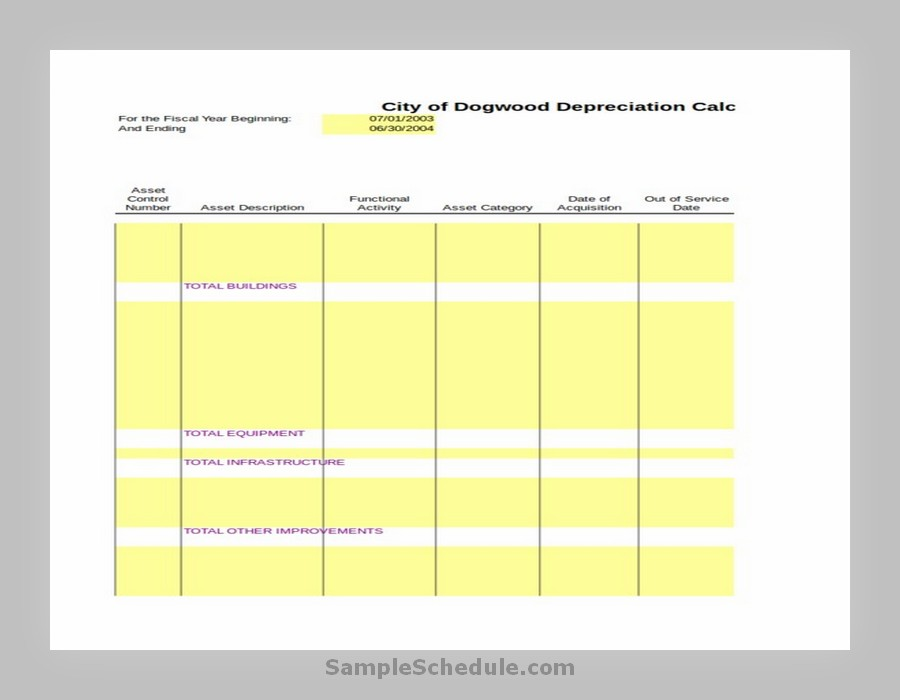Blank Depreciation Schedule WorksheetCapital Asset Depreciation Schedule Template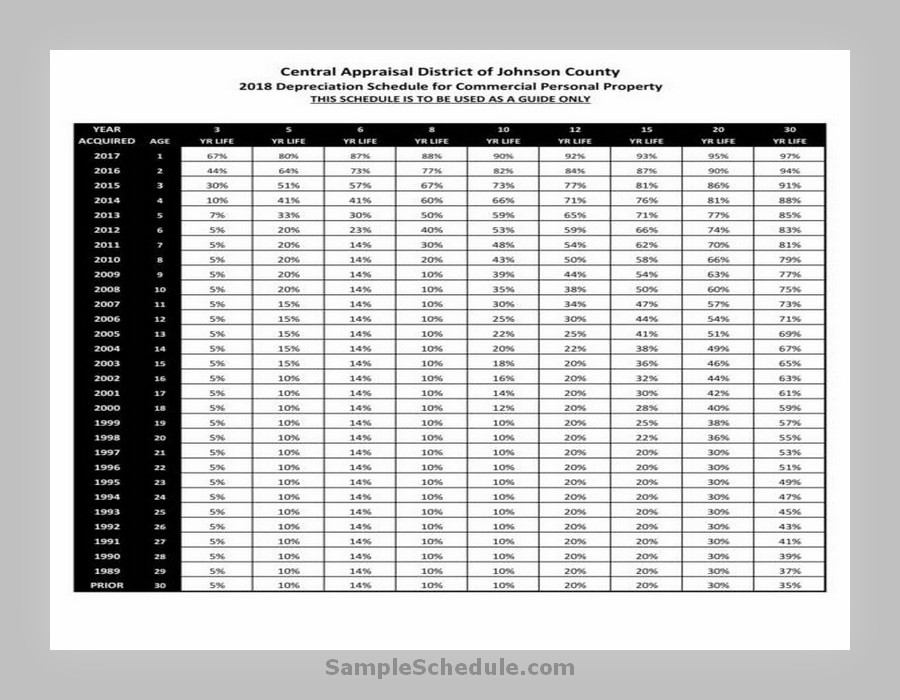Commercial Property Depreciation Schedule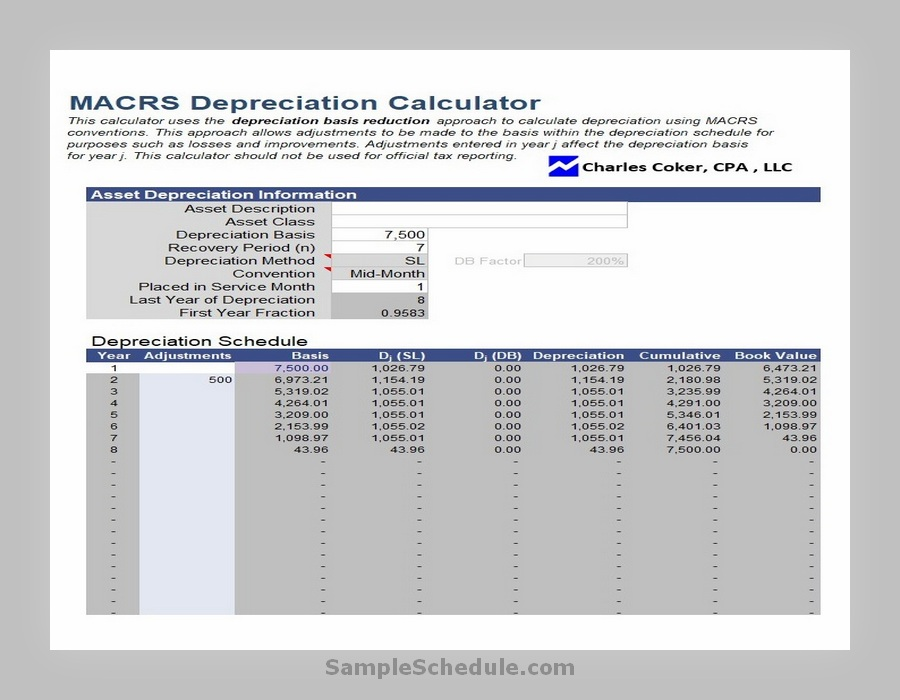Depreciation Calculator Schedule Template